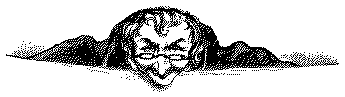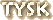# Mental Flexibility Test II

An alert reader and fan of such challenges clued me in to the following "test". I found this not only longer than my original
Mental Flexibility Test, but also more devious with the clues.
Only the brave will tackle this quiz before honing their wits on the first - shorter - one. Have fun!This test does not measure your intelligence, your fluency with words, and certainly not your mathematical ability. It will, however, give you some gauge of your mental flexibility and creativity. It has been found that few people could solve more than half on the first day. Many reported getting answers long after the test had been set aside, at unexpected moments when their minds were relaxed; and some reported solving questions over a period of several days.

Take this as a personal challenge.

Example: 16 = O in a P Answer: 16 = ounces in a pound

 1) 26 L. of the A. 2) 7 W. of the A. W. 3) 1,001 A. N. 4) 12 S. of the Z. 5) 54 C. in a D. (with J.) 6) 9 P. in the S. S. 7) 88 P. K. 8) 13 S. on the A. F. 9) 1 D. at a T. 10) 18 H. on a G. C. 11) 90 D. in a R. A. 12) 50 C. in a H. D. 13) 8 S. on a S. S. 14) 3 B. M. (S. H. T. R.) 15) 4 Q. in a G. 16) 24 H. in a D. 17) 1 W. on a U. 18) 5 D. in a Z. C. 19) 57 H. V. 20) 11 P. on a F. T. 21) 7 H. of R. 22) 101 D. 23) 64 S. on a C. B. 24) 13 C. in a S. 25) 10 L. I. 26) 20,000 L. U. T. S. 27) 13 O. C. 28) 12 K. of the R. T. 29) 13 in a B. D. 30) 66 B. of the B. 31) 10 D. in a D. 32) 15 M on a D. M. C. 33) 80 D. A. the W. 34) 24 B. B. in a P. 35) 7 B. for 7 B. 36) 36 I. in a Y. 37) 6 W. of H. the E. 38) 2,000 P. in a T. 39) 60 S. in a M. 40) 5 F. on the H. 41) 29 = D. in F. in a L. Y. 42) 3 P. for a F. G. in F. 43) 1,000 W. that a P. is W. 44) 56 S. of the D. of I. 45) 20 Y. that R. V. W. S. 46) 40 T (with A. B.) 47) 30 D. H. S. A. J. and N. 48) 32 D. F. at which W. F. 49) 10 A. in the B. of R. 50) 435 M. of the H. of R. 51) 31 I. C. F. at B.-R. 52) 200 D. for P. G. in M. 53) 2 T. D. (and a P. in a P. T.) 54) 4 H. of the A. 55) 40 D. and N. of the G. F. 56) 8 P. of S. in the E. L. 57) 9 I. in a B. G. 58) 1 R. A. in E. B. 59) 76 T. that L. the B. P. 60) 3 L. K. that L. T. M. 61) 4 S. on a V. 62) 5 T. on a C. (including S. in T.) 63) 6 P. on a P. T. 64) 7 Y. of B. L. for B. a M. 65) 2 G. for E. B. (in the B. B. S.) 66) 9 J. of the S. C. 67) 10 D. in a T. N. (including the A. C.) 68) 20 C. in a P. 69) 3 S. Y. O. at the O. B. G. 70) 1 C. Y. in K. A. C. 71) 3 W. from the G. in the B. 72) 20 Q. (A., V., or M.) 73) 7 D. with S. W. 74) 30 S. over T. 75) 8 D. a W. (in the B. S.) 76) 212 D. F. at which W. B. 77) 4 P. on M. R. 78) 10 D. in a R. of Q. 79) 48 S. in the C. U. S. 80) 7 C. on the E.

Scoring?
Unlike the earlier test no numerical "scores" have been assigned.
You are your own worst critic so you figure out how "flexible" you are!